Ex 3.6

Chapter 3 Class 10 Pair of Linear Equations in Two Variables
Serial order wise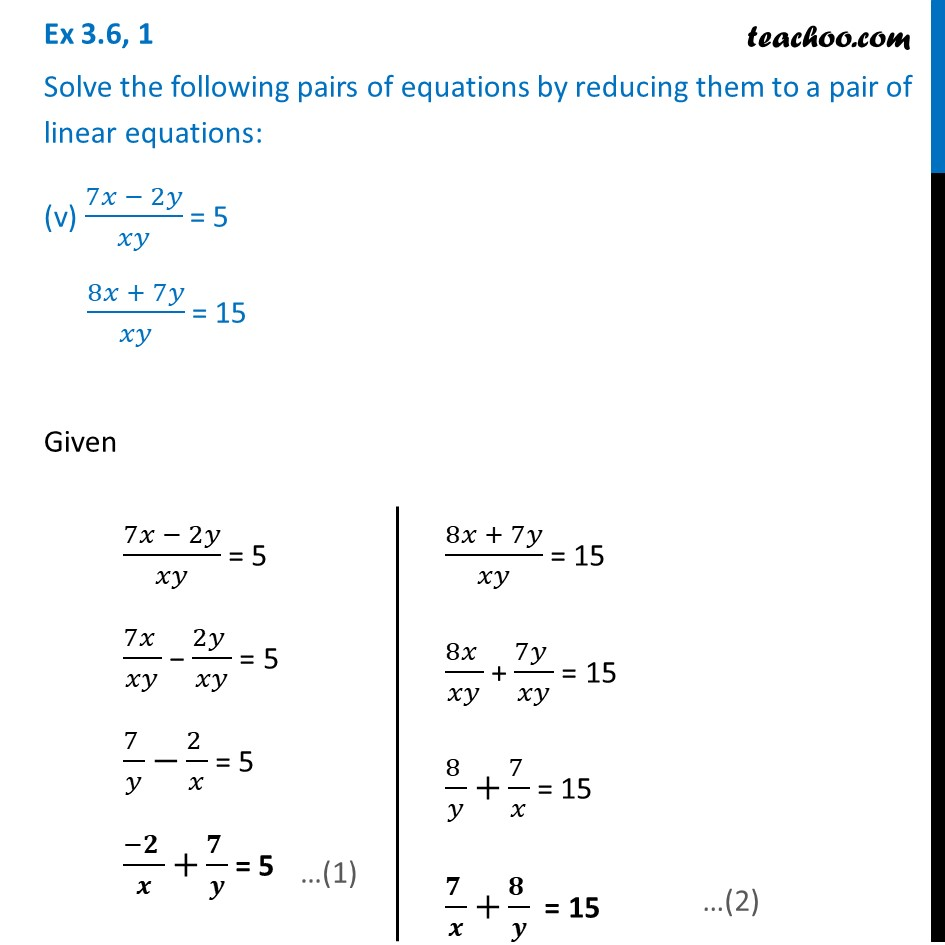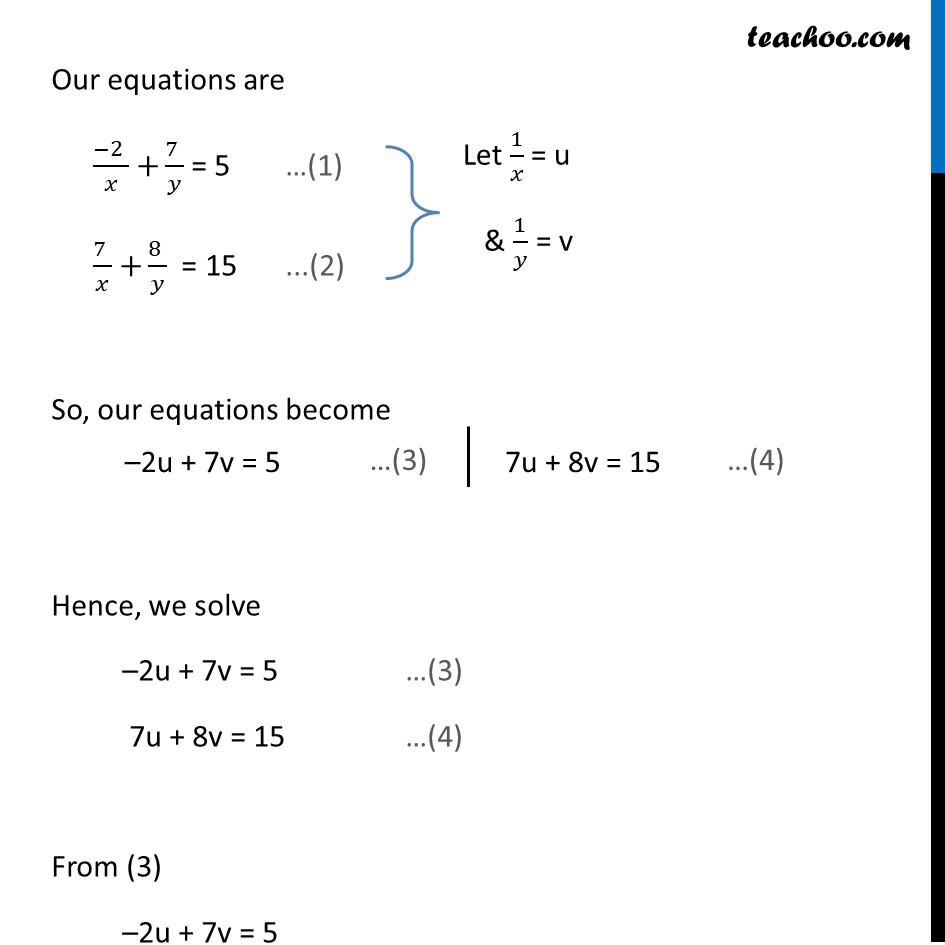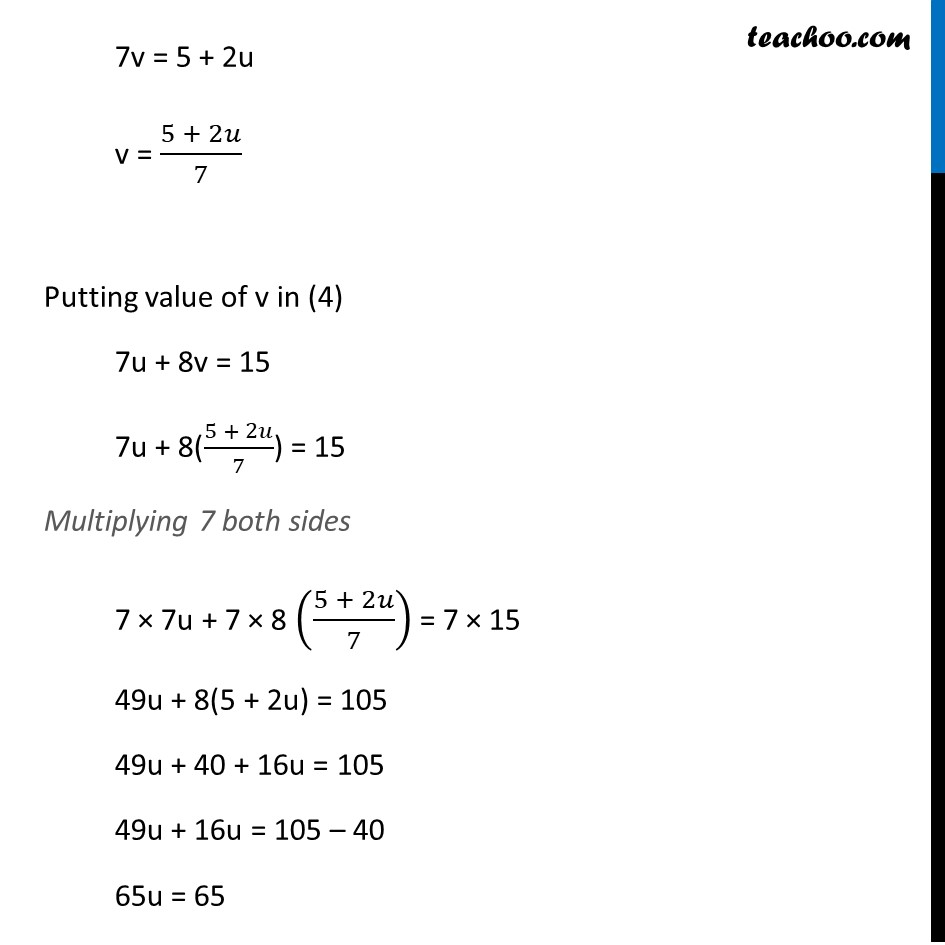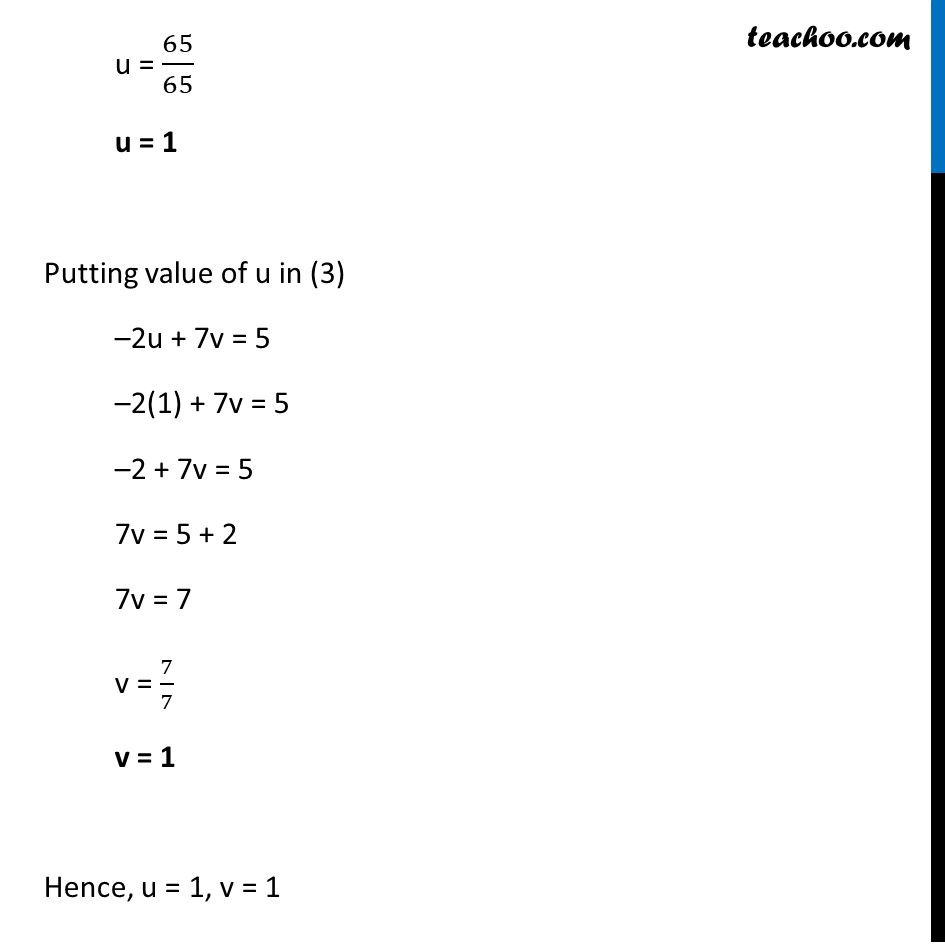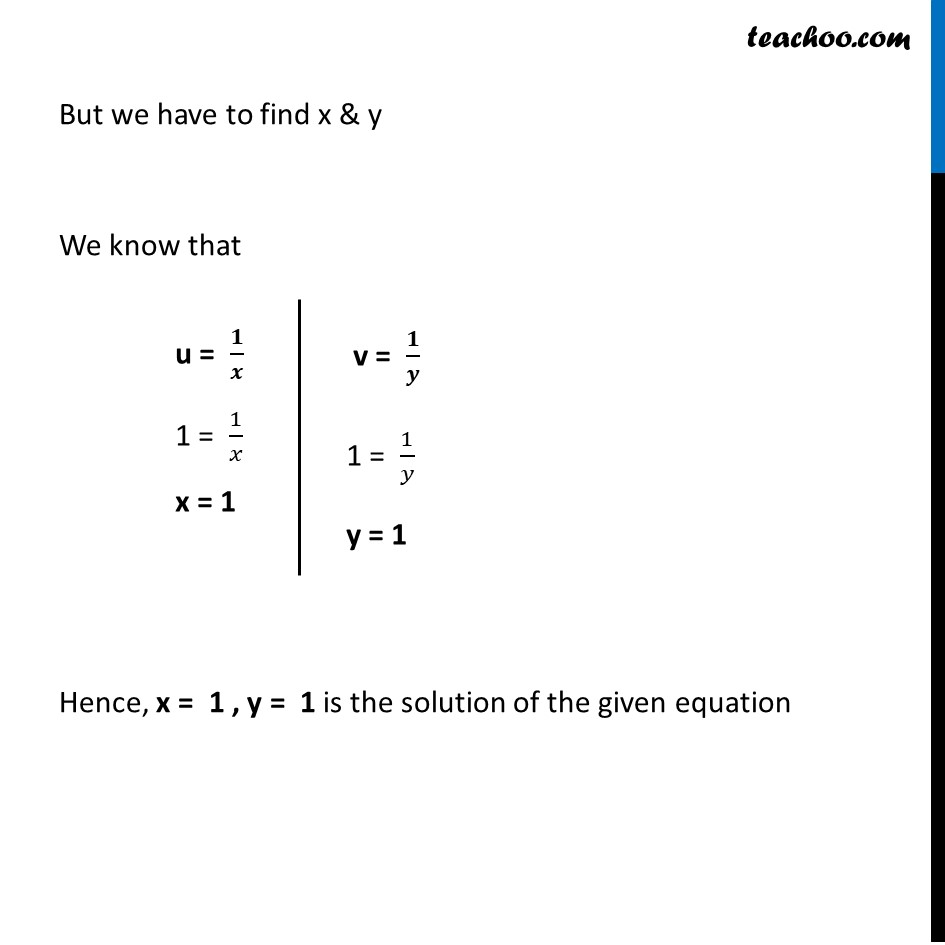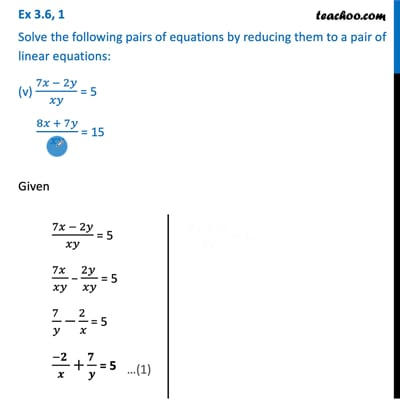This video is only available for Teachoo black users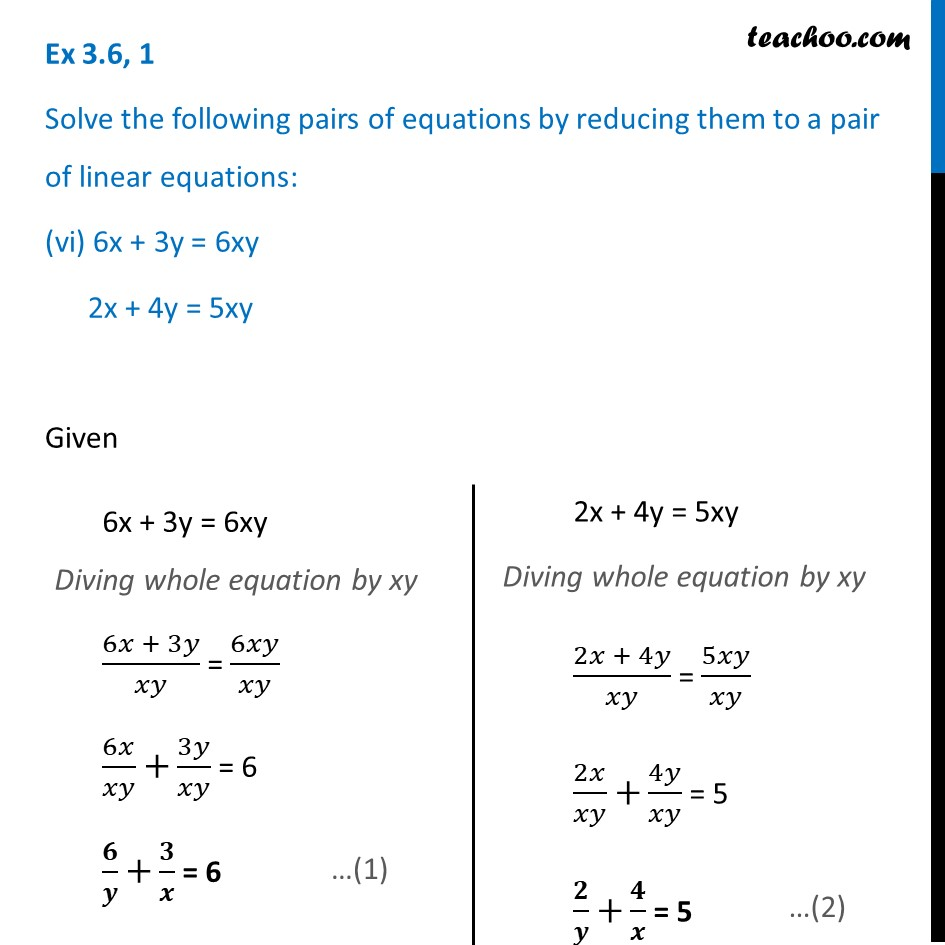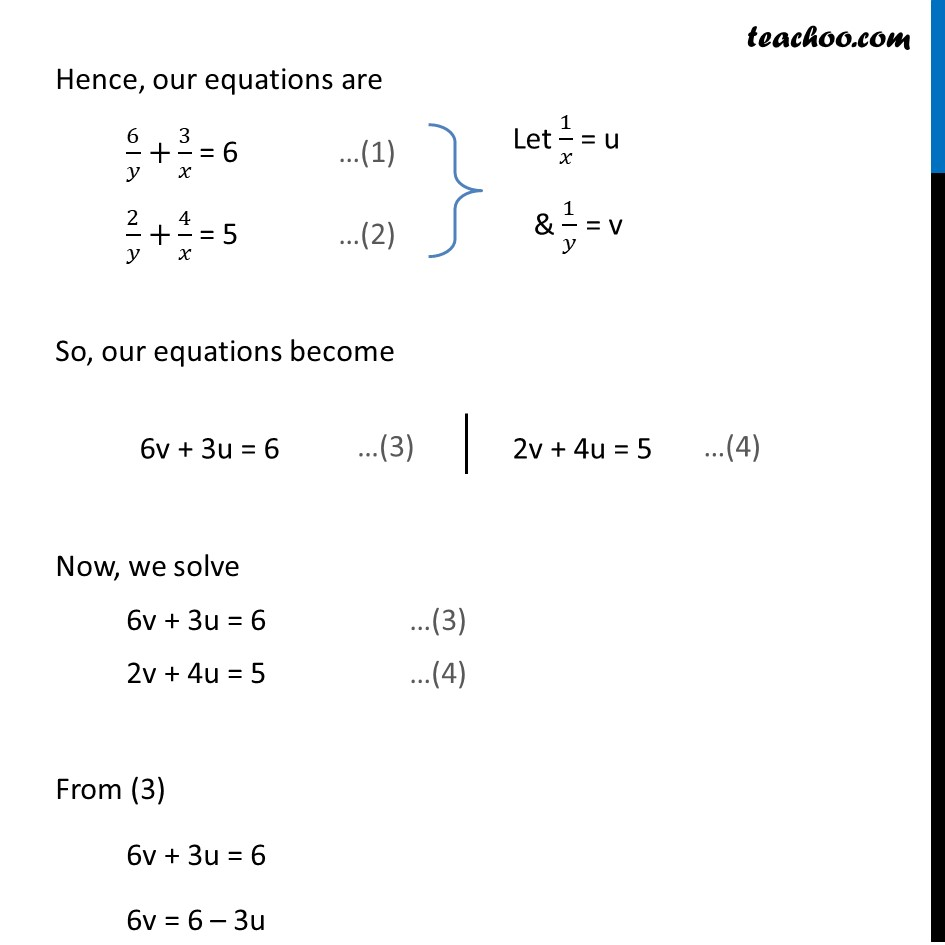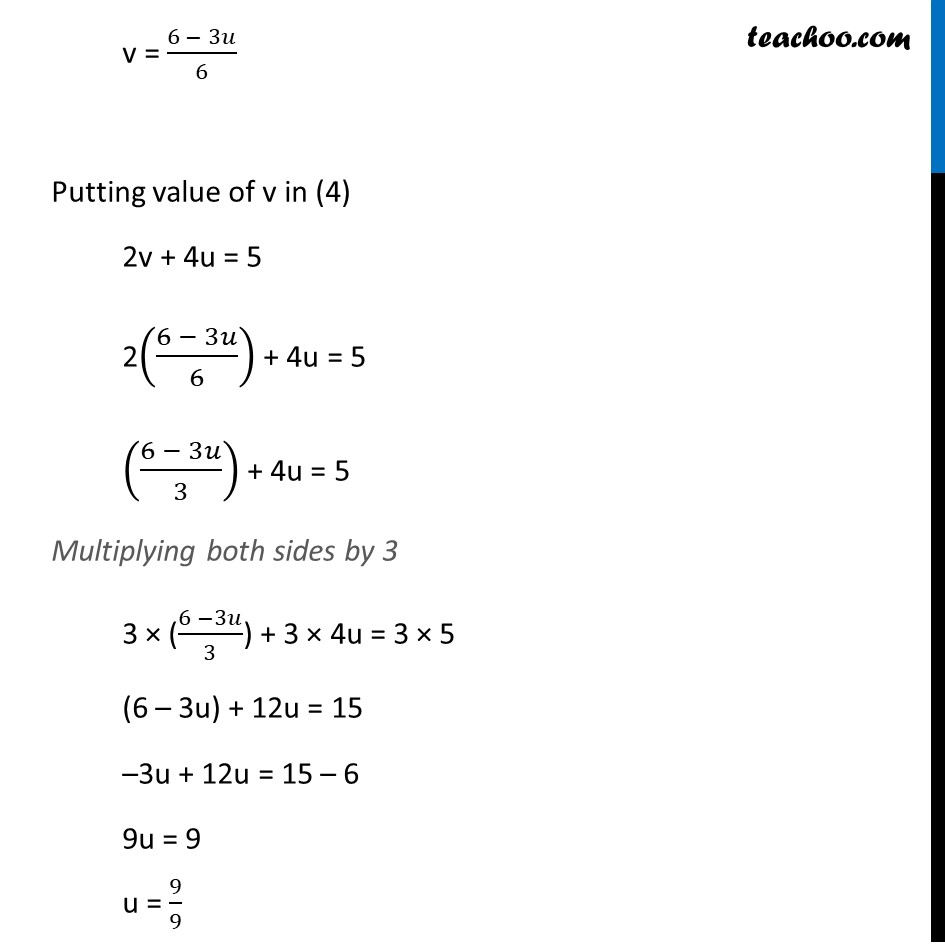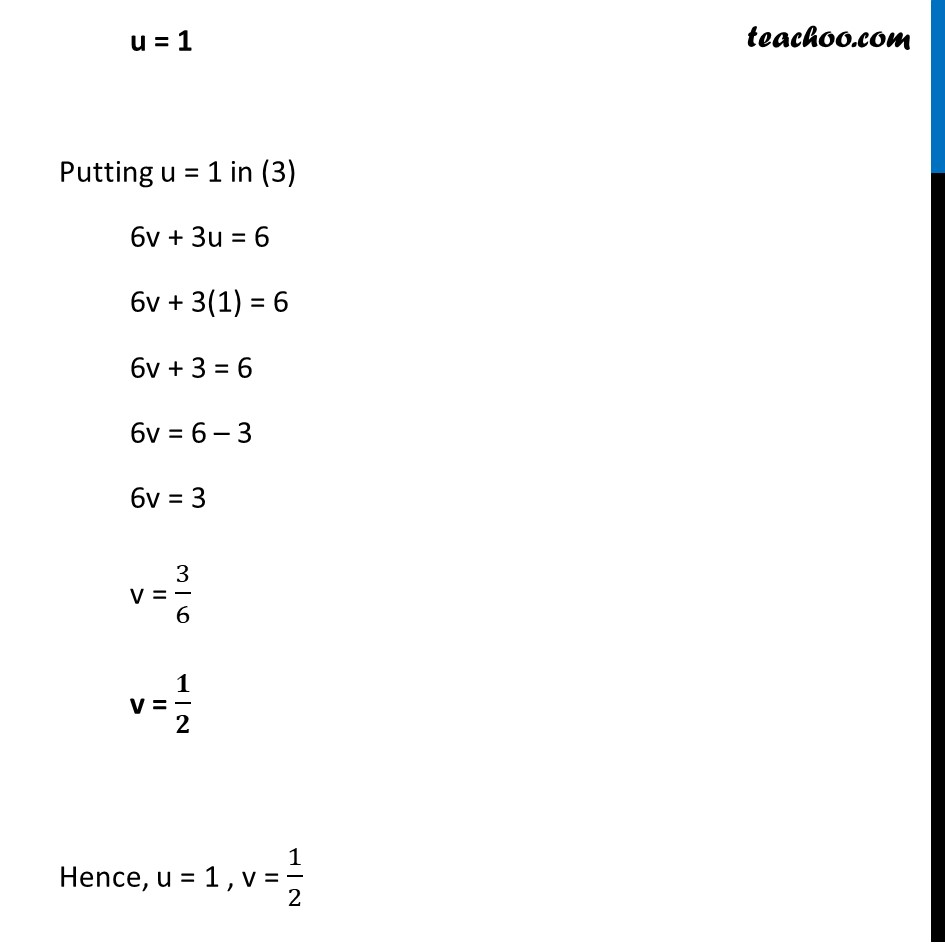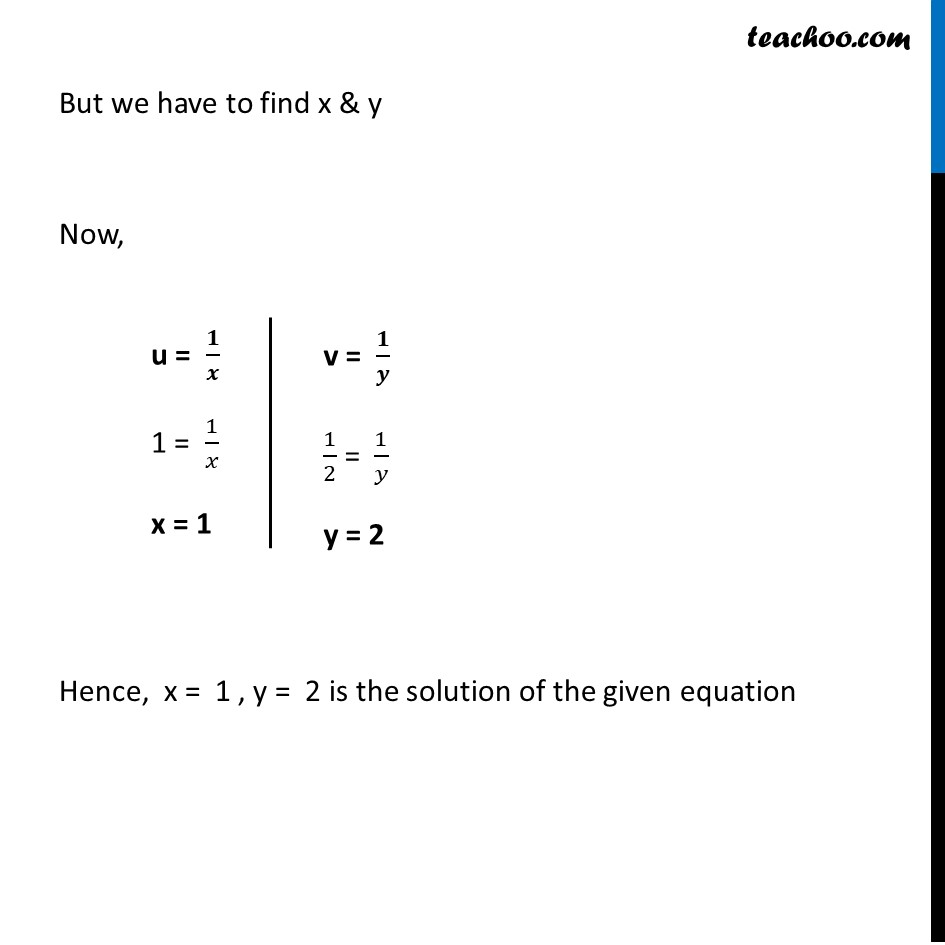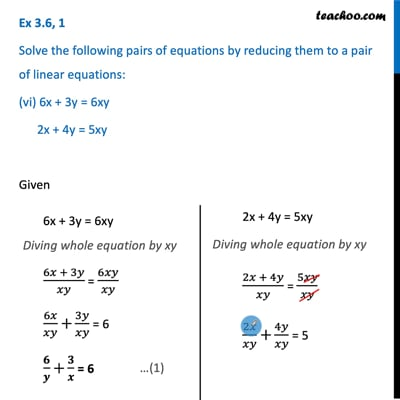This video is only available for Teachoo black users

Solve all your doubts with Teachoo Black (new monthly pack available now!)

### Transcript

Ex 3.6, 1 Solve the following pairs of equations by reducing them to a pair of linear equations: (v) (7𝑥 − 2𝑦)/𝑥𝑦 = 5 (8𝑥 + 7𝑦)/𝑥𝑦 = 15 Given (7𝑥 − 2𝑦)/𝑥𝑦 = 5 (7𝑥 )/𝑥𝑦 − (2𝑦 )/𝑥𝑦 = 5 (7 )/𝑦 −(2 )/𝑥 = 5 (−𝟐 )/𝒙 +(𝟕 )/𝒚 = 5 (8𝑥 + 7𝑦)/𝑥𝑦 = 15 (8𝑥 )/𝑥𝑦 + (7𝑦 )/𝑥𝑦 = 15 (8 )/𝑦 +(7 )/𝑥 = 15 (𝟕 )/𝒙 +(𝟖 )/𝒚 = 15 Our equations are (−2 )/𝑥 +(7 )/𝑦 = 5 …(1) (7 )/𝑥 +(8 )/𝑦 = 15 ...(2) So, our equations become –2u + 7v = 5 7u + 8v = 15 Hence, we solve –2u + 7v = 5 …(3) 7u + 8v = 15 …(4) From (3) –2u + 7v = 5 7v = 5 + 2u v = (5 + 2𝑢)/7 Putting value of v in (4) 7u + 8v = 15 7u + 8((5 + 2𝑢)/7) = 15 Multiplying 7 both sides 7 × 7u + 7 × 8 ((5 + 2𝑢)/7) = 7 × 15 49u + 8(5 + 2u) = 105 49u + 40 + 16u = 105 49u + 16u = 105 – 40 65u = 65 u = 65/65 u = 1 Putting value of u in (3) –2u + 7v = 5 –2(1) + 7v = 5 –2 + 7v = 5 7v = 5 + 2 7v = 7 v = 7/7 v = 1 Hence, u = 1, v = 1 But we have to find x & y We know that u = 𝟏/𝒙 1 = 1/𝑥 x = 1 v = 𝟏/𝒚 1 = 1/𝑦 y = 1 Hence, x = 1 , y = 1 is the solution of the given equation Ex 3.6, 1 Solve the following pairs of equations by reducing them to a pair of linear equations: (vi) 6x + 3y = 6xy 2x + 4y = 5xy Given 6x + 3y = 6xy Diving whole equation by xy (6𝑥 + 3𝑦)/𝑥𝑦 = 6𝑥𝑦/𝑥𝑦 6𝑥/𝑥𝑦 +3𝑦/𝑥𝑦 = 6 𝟔/𝒚 +𝟑/𝒙 = 6 2x + 4y = 5xy Diving whole equation by xy (2𝑥 + 4𝑦)/𝑥𝑦 = 5𝑥𝑦/𝑥𝑦 2𝑥/𝑥𝑦 +4𝑦/𝑥𝑦 = 5 𝟐/𝒚 +𝟒/𝒙 = 5 Hence, our equations are 6/𝑦 +3/𝑥 = 6 …(1) 2/𝑦 +4/𝑥 = 5 …(2) So, our equations become 6v + 3u = 6 2v + 4u = 5 Now, we solve 6v + 3u = 6 …(3) 2v + 4u = 5 …(4) From (3) 6v + 3u = 6 6v = 6 – 3u v = (6 − 3𝑢)/6 Putting value of v in (4) 2v + 4u = 5 2((6 − 3𝑢)/6) + 4u = 5 ((6 − 3𝑢)/3) + 4u = 5 Multiplying both sides by 3 3 × ((6 −3𝑢)/3) + 3 × 4u = 3 × 5 (6 – 3u) + 12u = 15 –3u + 12u = 15 – 6 9u = 9 u = 9/9 u = 1 Putting u = 1 in (3) 6v + 3u = 6 6v + 3(1) = 6 6v + 3 = 6 6v = 6 – 3 6v = 3 v = 3/6 v = 𝟏/𝟐 Hence, u = 1 , v = 1/2 But we have to find x & y Now, u = 𝟏/𝒙 1 = 1/𝑥 x = 1 v = 𝟏/𝒚 1/2 = 1/𝑦 y = 2 Hence, x = 1 , y = 2 is the solution of the given equation